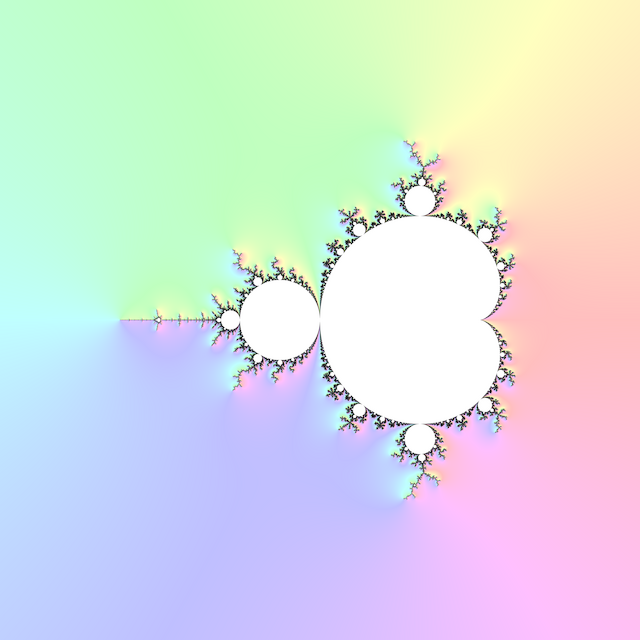\begin{aligned} v &= \left(\begin{matrix}\mu - \mu_x \\ \mu - \mu_y \\ s\end{matrix}\right) \\ g &= \frac{v_3}{\left|v\right|} \end{aligned}

where $$\mu_x$$ and $$\mu_y$$ are the continuous dwells of neighbouring pixels to the above and left of the pixel under consideration, and $$s > 0$$ is a parameter that controls the strength of the effect. $$0 \le g \le 1$$ colours the boundary of the set where iteration count goes up increasingly quickly. Define $$\mu$$ to be large and negative for pixels that didn’t escape.

Faking distance estimate colouring (2014) also describes using $$\tan^{-1} \frac{v_2}{v_1}$$ as hue for a rainbow colouring of dwell slope.

## # 1 C99 Code

#include <complex.h>
#include <math.h>

const double pi = 3.141592653589793;